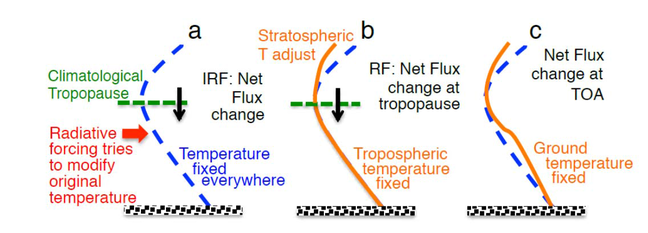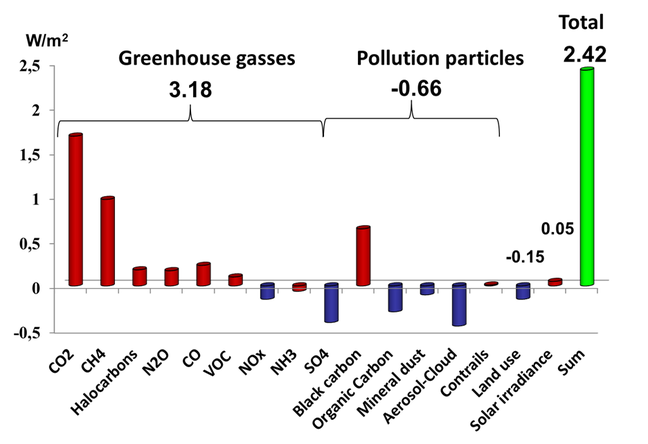## Want to keep learning?

This content is taken from the University of Bergen & Bjerknes Centre for Climate Research's online course, Causes of Climate Change. Join the course to learn more.
1.10

## University of BergenA radiative forcing is an energy imbalance imposed on the climate system either externally or by human activities (examples: changes in solar energy output, volcanic emissions, deliberate land modification, anthropogenic emissions of greenhouse gases, aerosols, and their precursors). It is usually expressed in watts per square meter ($$W/m^2$$) averaged over a particular period of time. A radiative forcing is something that can usually not be observed, but provides a simple quantitative basis for comparing the response in global mean temperature to different imposed agents.

Radiative forcing is defined as a radiative response to the forcing agent (for example $$CO_2$$), more quickly than the temperature can change substantially. Alternative definitions of radiative forcing have been developed in the literature and there are currently three slightly different forcing concepts in use: Instantaneous radiative forcing, stratospherically adjusted radiative forcing and effective radiative forcing.

The instantaneous radiative forcing (IRF): This is the radiative forcing defined as the instantaneous change in energy flux at the tropopause (around 15 km up in the atmosphere) resulting from a change in a component external to the climate system (for example man‐made $$CO_2$$ changes).

Stratospherically adjusted radiative forcing (RF): Many applications use an “adjusted” radiative forcing in which the stratosphere (the part of the atmosphere between 15 and 50km) is allowed to relax to the thermal steady state (for example adjust to the new $$CO_2$$ concentration) , leaving only energy imbalance in the Earth and troposphere (lowest 15 km of the atmosphere) system, which is most relevant to surface temperature change. Once the stratosphere has been allowed to adjust to a forcing, the change in energy flux at the tropopause is equivalent to that at the top of the atmosphere (TOA), which is where radiative forcings are commonly reported.Figure 1: Calculation of a) instantaneous radiative forcing where the atmospheric temperatures are held fixed when a radiative agent is imposed. b) Stratospherically adjusted radiative forcing where stratospheric temperatures are allowed to adjust and c) calculations where only the surface temperature is fixed to get the effective radiative forcing. Figure taken from IPCC AR5, WGI, chapter 8.

In many cases, such as for well mixed greenhouse gasses effective radiative forcing, stratospherically adjusted radiative forcing and instantaneous radiative forcing are nearly equal. However there are exceptions such as aerosols absorbing solar radiation for example black carbon where the ERF may deviate substantially from the RF.

## Historical changes in radiative forcings

Human activity has caused a variety of changes in different forcing agents in the atmosphere or land surface. The below figure summarize the radiative forcing for the period 1750–2011 based on emitted compounds.Figure 2: Radiative forcing ($$W/m^2$$) for the period 1750–2011 based on emitted compounds (gases, aerosols or aerosol precursors) or other changes. Positive values indicate increased radiative forcing, negative reduced radiative forcing. Values taken from IPCC AR5, WG1, Supplementary Material Tables 8.SM.6 and 8.SM.7.

## Measuring the effect of different radiative forcings

### Global‐warming potential (GWP)

Global‐warming potential (GWP) is a relative measure of the radiative forcing following a pulse emission of 1 kg of a given well‐mixed greenhouse gas in the present‐day atmosphere integrated over a chosen time horizon (commonly 20 or 100 years), relative to that of 1kg carbon dioxide. Thus, the GWP represents the combined effect of the differing times these gases remain in the atmosphere and their relative effectiveness in absorbing outgoing terrestrial radiation.

$$GWP(x) = \frac {\int\limits_{t=0}^{t=t_h} a_x\left\vert x(t) \right\vert dt }{\int\limits_{t=0}^{t=t_h} a_r\left\vert r(t) \right\vert dt}$$

where $$tk$$ is the time horizon over which the calculation is considered; $$ax$$ is the radiative efficiency due to a unit increase in atmospheric abundance of the substance (i.e., $$W/(m^2 kg)$$) and $$[x(t)]$$ is the time‐dependent decay in abundance of the substance following an instantaneous release of it at time $$t=0$$. The decay is often exponential and dependent on the lifetime of the substance. The denominator contains the corresponding quantities for the reference gas (i.e. $$CO_2$$). The radiative efficiencies ax and ar are not necessarily constant over time. While the absorption of infrared radiation by many greenhouse gases varies linearly with their abundance, a few important ones display non‐linear behaviour for current and likely future abundances (e.g., $$CO_2$$, $$CH_4$$, and $$N_2O$$).

One criticism of GWP relates to the fact that, despite its name, it does not represent the impact of gas emissions on temperature. The GWP uses the time‐integrated radiative forcing and this does not give a unique indication of the effect of pulse emissions on temperature, because of large differences in the time constants of the various greenhouse gases. Although a strong greenhouse gas with a short lifetime could have the same GWP as a weaker greenhouse gas with a longer lifetime, identical (in mass terms) pulse emissions of the two gases could cause a different temperature change at a given time.

It is also possible to calculate an indirect Global‐warming potential which is the indirect radiative effects which include the direct effects of degradation products or the radiative effects of changes in concentrations of greenhouse gases caused by the presence of the emitted gas or its degradation products. For example through the CO and VOCs effect on ozone and methane (which are greenhouse gases) formation they can be viewed as having indirect Global‐warming potentials. Uncertainties for the indirect GWPs are generally much higher than for the direct GWPs and will in many cases depend on the location and time of the emissions. Thus, the usefulness of the global mean indirect GWPs can be limited.

### The Global Temperature Potential (GTP)

The Global Temperature Potential (GTP) is defined as the ratio between the global mean surface temperature change at a given future time horizon (H) following an emission (pulse or sustained) of a compound x relative to a reference gas $$r$$ (e.g. $$CO_2$$):

$$GTP^H(x) =\frac{\Delta T^{t=H}(x)}{\Delta T^{t=H}(r)}$$

where $$\triangle T^{t=H}(x)$$ denotes the global mean surface temperature change after H years following an emission of compound x. The GTPs do not require simulations with a fully coupled climate model (AOGCM), but are given as transparent and simple formulas that employ a small number of input parameters required for calculation. Note that while the GWP is an integral quantity over the time horizon (i.e., the contribution of the RF at the beginning and end of the time horizon is exactly equal), the GTP uses the temperature change at time H (i.e., RF closer to time H contributes relatively more). The GTP metric requires knowledge of the same parameters as the GWP metric (radiative efficiency and lifetimes), but in addition, the response times for the climate system must be known, in particular if the lifetime of component x is very different from the lifetime of the reference gas. The fact that two greenhouse gases with the same radiative forcing do not necessarily lead to the same temperature increase (different climate efficacies) can easily be incorporated into the GTP metric. Due to the inclusion of the response times for the climate system, the GTP values for pulse emissions of gases with shorter lifetimes than the reference gas will be lower than the corresponding GWP values. There is a near equivalence between the GTP for sustained emission changes and the pulse GWP. The GTP metric has the potential advantage over GWP that it is more directly related to surface temperature change. However, two different greenhouse gasses with equal GTPs (same temperature change at time H) may not at specific times between 0 and H have the same effect on temperature. In other words, two different emissions that give the same temperature effect at a chosen time H can have different paths. That means that total climate impact of these two gases might be very different. The GTP will not reflect these differences.